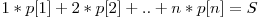# Hill Climbing shortlistCosmin Negruseri
23 iunie 2015

Here are a few problems that involve hill climbing or some form of local search. Feel free to suggest others and to discuss solutions.

1. You are given a list of A of n points with integer coordinates in the 2d plane. Find another point P(x, y) such that the sum of square distances from the points in A to P is minimum.
2. You are given a list of A of n points with integer coordinates in the 3d plane. Find another point P(x, y) such that the maximum of the Manhattan distances from the points in A to P is minimum. (the manhattan distance between (x1, y1, z1) and (x2, y2, z2) is |x1 - x2| + |y1 - y2| + |z1 - z2|)
3. You are given a list of A of n points with integer coordinates in the 2d plane. Find another point P(x, y) such that the sum of euclidean distances from the points in A to P is minimum.
4. You are given a convex polygon P with n vertices, find out the radius of the largest inscribed circle in that polygon..
5. You are given a list A of n points in the plane, find out the minimum enclosing circle.
6. Given a number n, find out a placement of n queens on an nxn chessboard such that they don’t attack each other.
7. Given n (n <= 1000) and S integers, find out a permutation p such that.
8. 2n knights have to sit around a roundtable. Each knight is friends with n + 1 other knights. Find a seating arrangement such that each knight is placed between two friends.
9. Given two line segments in 3d space find the minimum distance between them.
10. A party of n people is too large and has to be split to two tables. Given that each of the people has at most three enemies in the group, find a seating arrangement such that each person sits at a table with at most one of his enemies.
11. Given a set A of n black points and a set B of n white points (no three points are collinear), join each point in A with one point in B respectively such that no two segments will intersect.
12. Given a set A with n points and a set B with m points, find a line that separates the two sets.
13. Given two points A and B and a circle C, find a point D on C such that AD + DB is minimized.
14. Given a rectangle R find a list L of n points in it such that the sum of distances between all pairs of points in L is maximized.
Categorii:
remote content